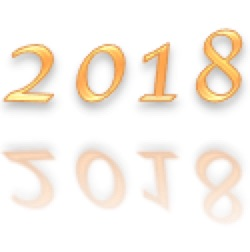You can be a creative mathematician, you can be someone who has your own mathematical ideas.

Find your own interesting facts about 2018 and calculations that have the answer 2018. For example did you know that 2018 is the product of two prime numbers?

You might think of calculations like: 2 x 1009 = 2018 or more complicated ones …
$$\frac{4036}{2} = 2018$$
$$2^{11}-2^5 +2=2018 \\ 3\times6! – 5! – 4! +2! = 2018$$

What different questions can you find with 2018 as the answer?

Compare your questions with other people’s.

You might like to use a calculator.

Perhaps you can make up one that is easy, one that is harder and one that is very hard.

As an extra challenge: divide 1009 by the prime numbers from 2 to 31. Try it using a calculator. Can you explain why this is sufficient to show that 1009 is a prime number?

What interesting facts can you find about the year that you were born?